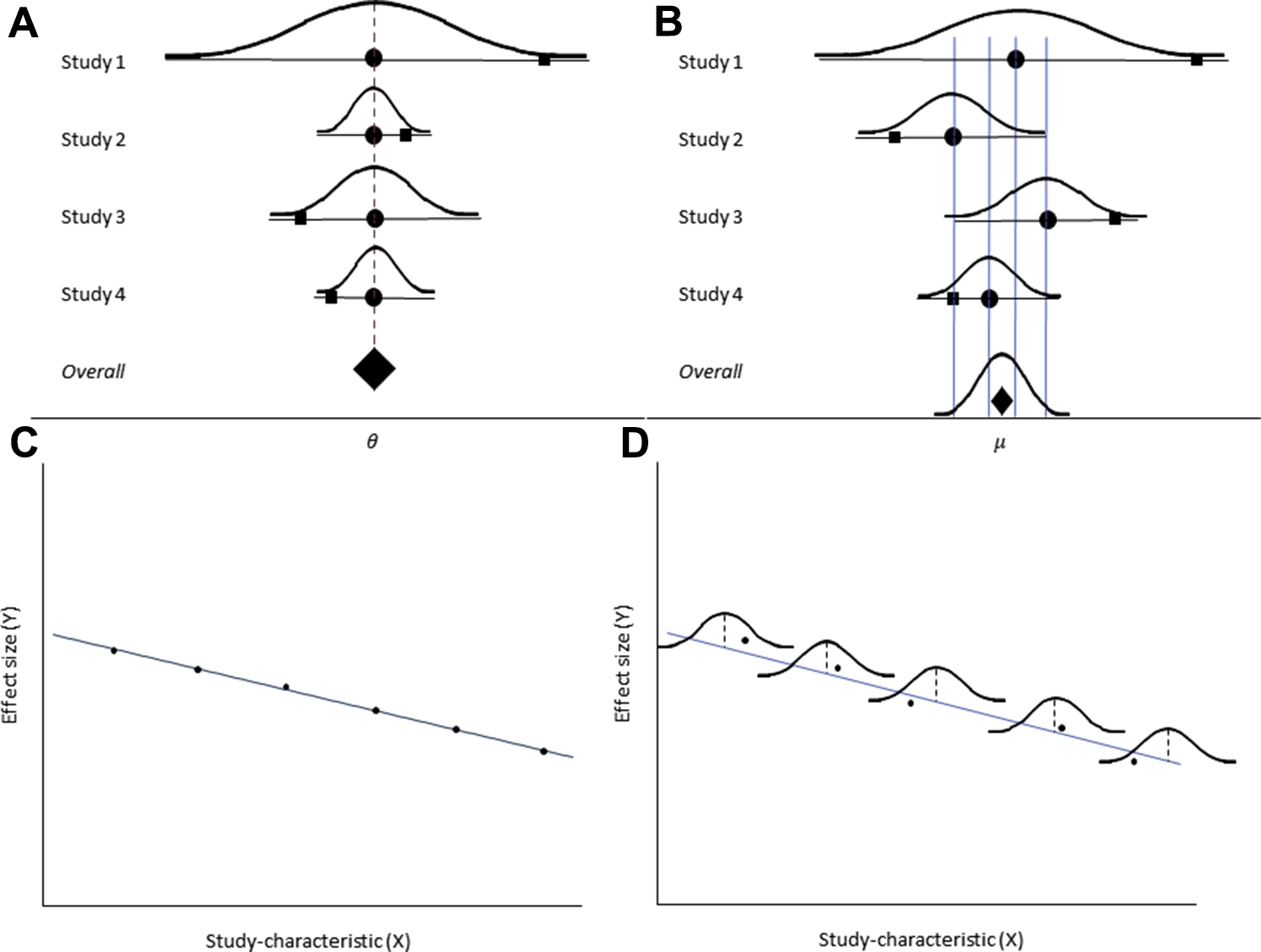# Fixed-effect versus random-effects model in meta-regression analysis

In earlier articles of this column, , we discussed the differences between the fixed-effect and the random-effects model for meta-analysis. Under a fixed-effect model, we assume a common true effect size across all studies, and we estimate this common effect size (known as summary effect size), whereas under the random-effects model, we assume a normal distribution of true effect sizes and estimate the mean (also known as summary effect size) and the variance (known as heterogeneity) of this distribution. The differences in these 2 models are illustrated by forest plots in Figures A and B . The effect sizes have been synthesized under a fixed-effect model ( Figs A and C ) and random-effects model ( Figs B and D ).Fig

In the articles on subgroup analysis, , we explained how these 2 models apply in a subgroup analysis. Under a fixed-effect model, we assume a common true effect size across all studies in each subgroup and estimate these subgroup-specific common effect sizes. Under the random-effects model, we assume a normal distribution of the true effect sizes in each subgroup and estimate the mean and the variance of this distribution in each subgroup. A similar approach of the models also holds in a meta-regression analysis. Under a fixed-effect model, the true effect size coincides with the corresponding predicted effect size (ie, the value obtained by using the linear equation that defines a typical regression model), whereas under a random-effects model, there is a distribution of effect sizes about each predicted value; namely, the true effect size can fall anywhere in the range of the distribution centered on the corresponding predicted effect size. Figures C and D , clarify the idea of fixed-effect and random-effects models in a meta-regression analysis. A fixed-effect meta-regression model ( Fig C ) and a random-effects meta-regression model ( Fig D ) have been implemented.

In addition, the different assumptions for fixed-effect and random-effects models reflect different definitions of the variance (which results in different computations of the meta-analysis results because of different weighting schemes) and different determinations of the null hypothesis of no linear association. Under a fixed-effect model, the source of error is only the within-study variation, and the null hypothesis states that the common true effect size is not associated with the covariate of interest. By contrast, under a random-effects model, the sources of error are both within-study and between-study variance, and the null hypothesis states that the mean of the true effect sizes is not associated with the covariate of interest.

The meaning of the heterogeneity parameter τ 2 differs among a random-effects meta-analysis model, a random-effects subgroup analysis, and a random-effects meta-regression model. In a meta-analysis, τ 2 reflects the dispersion of the true effect size across the studies included in the meta-analysis, and we estimate the T 2 for this set of studies. In a subgroup analysis, τ 2 reflects the dispersion of the true effect size across the studies included in each subgroup, and we estimate the T 2 within each subgroup. For example, if we distinguish 2 sets of trials on the basis of the trial design (randomized controlled trials and controlled clinical trials), we need to calculate a τ 2 for the set of randomized controlled trials and a τ 2 for the set of controlled clinical trials to quantify the statistical heterogeneity observed in each subgroup. Finally, in a meta-regression, τ 2 reflects the dispersion of the true effect size across the studies that have the same covariate value (and the same predicted effect size—the point on the prediction line). For example, if we are interested in investigating the association between the risk ratio and the total sample size, the parameter τ 2 will reflect the residual statistical heterogeneity, namely, the statistical heterogeneity that remains after adjusting for the variation of the risk ratio due to different total sample size across the trials. The residual statistical heterogeneity may be attributed to trial and/or patient characteristics that may be associated with the outcome but we have not included in the meta-regression model.

As already mentioned in previous sections, the selection of the model depends on our assumptions about the similarity of the chosen studies in terms of their clinical characteristics, quality, design, and conduct. , , If there are strong reasons to believe that the studies are identical for all intents and purposes, then a fixed-effect meta-regression model is an appropriate choice. However, the assumptions that underlie the fixed-effect model do not usually hold. For instance, it is implausible to consider identical studies published in different years or studies conducted in different geographic settings. Not only the year of publication but also the geographic setting, among other study characteristics, can have an impact on the distribution of the true effects to some degree. Therefore, a random-effects model is the appropriate model because it captures the distribution of the true effect sizes.

## The R 2 index: the proportion of variance explained

We have already introduced this intuitive index in the article on subgroup analysis of this column. , This index can be applied in meta-regression analysis, as well. It quantifies the proportion of variance of the true effect sizes (heterogeneity) that can be explained by the covariates, and it is defined as a ratio of the explained heterogeneity to the total heterogeneity :

<SPAN role=presentation tabIndex=0 id=MathJax-Element-1-Frame class=MathJax style="POSITION: relative" data-mathml='R2=Texplained2Ttotal2′>𝑅2=𝑇2explained𝑇2𝑡𝑜𝑡𝑎𝑙R2=Texplained2Ttotal2
R2=Texplained2Ttotal2
Only gold members can continue reading. Log In or Register to continue

Jan 9, 2021 | Posted by in Orthodontics | Comments Off on Fixed-effect versus random-effects model in meta-regression analysis
Premium Wordpress Themes by UFO Themes